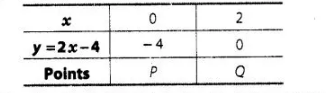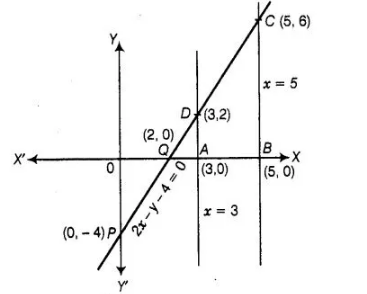# Draw the graphs of the equations x = 3,

Question:

Draw the graphs of the equations x = 3, x = 5 and 2x – y – 4 = 0. Also find the area of the quadrilateral formed by the lines and the X-axis.

Solution:

Given equation of lines 2x – y – 4 = 0, x = 3 and x = 5 Table for line 2x – y – 4 = 0 Also

find the area of the quadrilateral formed by the lines and the X-axis.Draw the points $P(0,-4)$ and $Q(2,0)$ and join these points and form a line $P Q$ also draw the lines $x=3$ and $x=5$.$\therefore$ Area of quadrilateral $A B C D=\frac{1}{2} \times$ distance between parallel lines $(A B) \times(A D+B C)$

[since, quadrilateral $A B C D$ is a trapezium]

$=\frac{1}{2} \times 2 \times(6+2)$

$[\because A B=O B-O A=5-3=2, A D=2$ and $B C=6]$

$=8 \mathrm{sq}$ units

Hence, the required area of the quadrilateral formed by the lines and the X-axis is 8 sq units.